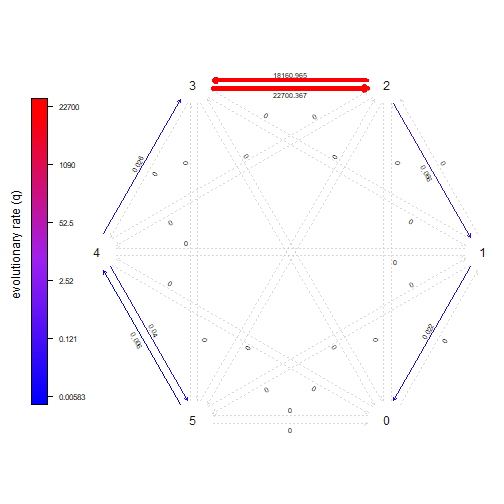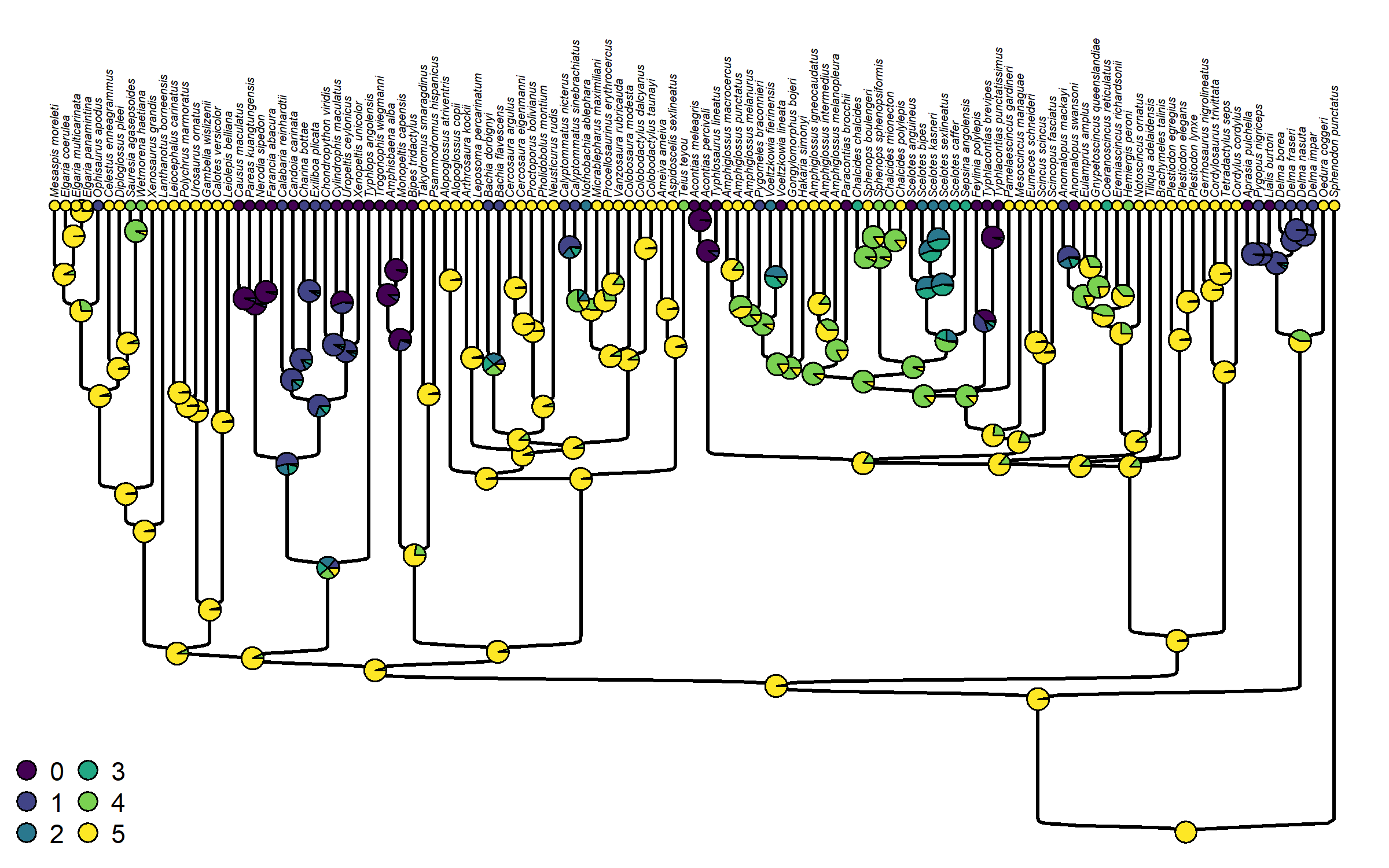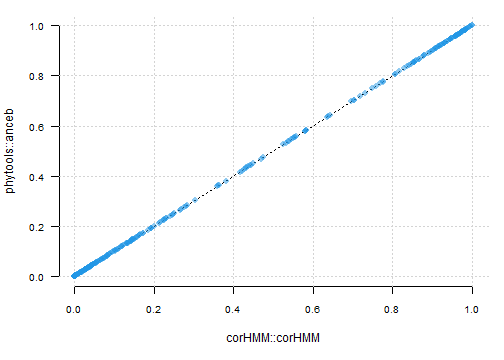## Thursday, March 23, 2023

### Generic method for marginal ancestral state estimation in phytools

I just added marginal ancestral state reconstruction to phytools as a generic method, `anceb` (for “empirical Bayes” ancestral state estimation).

The way this method works is that it takes a fitted Mk model as input, and then it proceeds to compute our ancestral character states (marginal scaled likelihoods) for all the internal nodes of the phylogeny.

To try it out, we should first update phytoools from GitHub (which can be done, for example, using the CRAN package remotes) – and then load it.

``````library(phytools)
packageVersion("phytools")
``````
``````##  '1.6.0'
``````

Next, to illustrate the method I’ll use a phylogeny of squamate reptiles and a count of digit number in the hindfoot, modified from Brandley et al. (2008). (We also use this dataset in our recent book, so readers can download it from the book website.)

Here, I’ll fit a bi-directional “ordered” model – with 5 loss rates (a different rate between each number of digits from 5 through 0) and 5 gain rates (ditto, but in reverse), for a total of 10 parameters in the fitted model.

In our book, we show that this model is not particularly well-supported by the data – especially compared to a “loss-only” model, but it creates a more interesting ancestral state reconstruction than the loss-only model, so that’s why I’m using it here. I’ll also fix the ancestral state at the global root to be 5 digits.

``````sqTree<-read.nexus(file="http://www.phytools.org/Rbook/6/squamate.tre")
row.names=1)
chk<-geiger::name.check(sqTree,sqData)
sqTree<-drop.tip(sqTree,chk\$tree_not_data)
toes<-setNames(sqData\$rear.toes,rownames(sqData))[sqTree\$tip.label]
ordered<-matrix(c(
0,6,0,0,0,0,
1,0,7,0,0,0,
0,2,0,8,0,0,
0,0,3,0,9,0,
0,0,0,4,0,10,
0,0,0,0,5,0),6,6,byrow=TRUE)
fitOrdered<-fitMk(sqTree,toes,model=ordered,
opt.method="optimParallel",rand_start=TRUE,
pi=c(0,0,0,0,0,1))
fitOrdered
``````
``````## Object of class "fitMk".
##
## Fitted (or set) value of Q:
##          0         1         2             3         4         5
## 0 0.000000  0.000000      0.00      0.000000  0.000000  0.000000
## 1 0.022285 -0.022285      0.00      0.000000  0.000000  0.000000
## 2 0.000000  0.066128 -18161.03  18160.964853  0.000000  0.000000
## 3 0.000000  0.000000  22700.37 -22700.366700  0.000000  0.000000
## 4 0.000000  0.000000      0.00      0.026326 -0.066346  0.040020
## 5 0.000000  0.000000      0.00      0.000000  0.005827 -0.005827
##
## Fitted (or set) value of pi:
## 0 1 2 3 4 5
## 0 0 0 0 0 1
## due to treating the root prior as (a) given.
##
## Log-likelihood: -114.77994
##
## Optimization method used was "optimParallel"
``````
``````plot(fitOrdered,width=TRUE,color=TRUE,
mar=rep(0.1,4))
``````One thing that readers might observe about the fitted model is that it involves very high estimated transition rates between character states 2 ⇄ 3. This probably doesn’t mean much – aside from perhaps indicating that if a lineage is in character state 3 it can very easily evolve to 2 (and perhaps vice versa) – and that the likelihood surface is probably quite flat from a high value to a very high value, as we found for our case.

Now to estimate ancestral states under this model all that we need to do is pass our fitted model object from `fitMk` directly to our generic method. (Later, this will also be true for other Mk model types – such as `fitpolyMk` and `fitHRM`.)

``````ordered_anc<-anceb(fitOrdered)
ordered_anc
``````
``````## Marginal ancestral character estimates:
##     0 1 2 3        4        5
## 120 0 0 0 0 0.000000 1.000000
## 121 0 0 0 0 0.025017 0.974983
## 122 0 0 0 0 0.025942 0.974058
## 123 0 0 0 0 0.041112 0.958888
## 124 0 0 0 0 0.073954 0.926046
## 125 0 0 0 0 0.063408 0.936592
## ...
##
## Log-likelihood = -114.77994
``````

Finally, let’s plot these marginal states onto the nodes of the tree.

``````sigmoidPhylogram(sqTree,direction="upwards",fsize=0.4,ftype="i",
offset=1)
cols<-setNames(viridisLite::viridis(n=6),0:5)
nodelabels(pie=ordered_anc\$ace,piecol=cols,cex=0.4)
tiplabels(pie=fitOrdered\$data[sqTree\$tip.label,],
piecol=cols,cex=0.2)
legend("bottomleft",legend=0:5,pch=21,pt.bg=cols,ncol=2,bty="n",
pt.cex=1.5,cex=0.9)
``````Great. We’re done!

As a sanity check of our implementation, why don’t we fit the same model using the corHMM package function `corHMM` by Beaulieu et al. This is the function that we trusted for marginal ancestral character estimation in our book.

``````rate.mat<-ordered
rate.mat[ordered==0]<-NA
fit_corhmm<-corHMM::corHMM(sqTree,
data.frame(Genus_sp=names(toes),toes=toes),
rate.cat=1,rate.mat=rate.mat,
root.p=c(0,0,0,0,0,1),node.states="marginal")
``````
``````## Warning in corHMM::corHMM(sqTree, data.frame(Genus_sp = names(toes), toes = toes), : Branch lengths of 0
## detected. Adding 1e-5 to these branches.
``````
``````## State distribution in data:
## States:	1	2	3	4	5	6
## Counts:	25	16	5	4	6	63
## Beginning thorough optimization search -- performing 0 random restarts
## Finished. Inferring ancestral states using marginal reconstruction.
``````
``````fit_corhmm
``````
``````##
## Fit
##       -lnL      AIC     AICc Rate.cat ntax
##  -114.7801 249.5602 251.5972        1  119
##
## Legend
##   1   2   3   4   5   6
## "0" "1" "2" "3" "4" "5"
##
## Rates
##            (1,R1)      (2,R1) (3,R1)      (4,R1)      (5,R1)     (6,R1)
## (1,R1)         NA 0.000000001     NA          NA          NA         NA
## (2,R1) 0.02228573          NA  1e-09          NA          NA         NA
## (3,R1)         NA 0.066177260     NA 80.01271308          NA         NA
## (4,R1)         NA          NA  1e+02          NA 0.000000001         NA
## (5,R1)         NA          NA     NA  0.02632766          NA 0.04001653
## (6,R1)         NA          NA     NA          NA 0.005826560         NA
##
## Arrived at a reliable solution
``````

Now let’s compare our marginal likelihoods from one to the other.

``````par(mar=c(5.1,4.1,1.1,1.1))
plot(NA,xlim=c(0,1),ylim=c(0,1),las=1,cex.axis=0.8,
xlab="corHMM::corHMM",ylab="phytools::anceb",bty="n")
grid()
lines(c(0,1),c(0,1),lty="dotted")
points(ordered_anc\$ace,fit_corhmm\$states,pch=16,
col=make.transparent(palette(),0.5),cex=1.2)
``````OK – well that’s no guarantee we’ve gotten things right, but it should lend us a tiny measure of satisfaction!